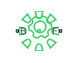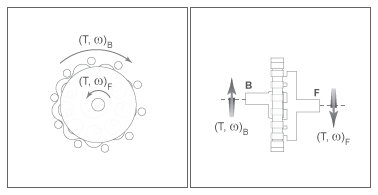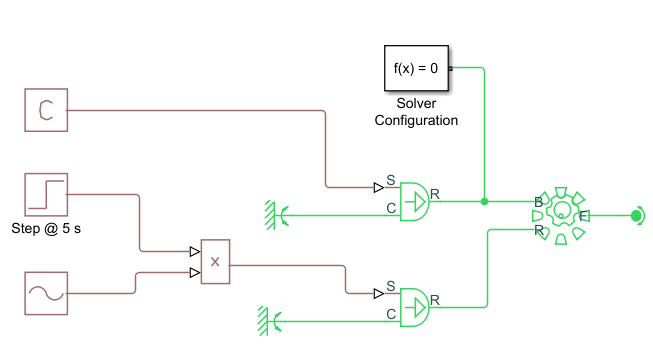# Cycloidal Drive

High-ratio speed reducer based on cycloidal disc motion

•Libraries:
Simscape / Driveline / Gears

## Description

The Cycloidal Drive block represents a compact, high-ratio, speed-reduction mechanism that contains four key components:

• A cycloidal disc

• An eccentric cam

• Ring-gear housing

• Pin rollers

The eccentric cam, which extends from the base shaft, sits inside the cycloidal disc. This disc meshes with the ring-gear housing. The pin rollers, which extend from the follower shaft, sit in matching holes on the cycloidal disc.During normal operation, the base shaft drives the eccentric cam. The cam spins inside the cycloidal disc, causing it to rotate in an eccentric pattern about an offset axis. As it moves, the cycloidal disc engages the internal teeth of the ring-gear housing. The internal meshing reverses the rotational velocity direction.

The pin rollers extending through the cycloidal disc holes transmit rotational motion to the follower shaft. This shaft spins counter to the base shaft at a very reduced speed. The high reduction ratio results from the near-equal cycloidal disc and ring gear tooth numbers. The block calculates the effective gear reduction ratio as

`$r=\frac{{n}_{R}-{n}_{C}}{{n}_{C}},$`

where:

• r is the gear reduction ratio.

• nR is the number of teeth on the ring gear.

• nC is the number of teeth on the cycloidal disc.

The gear reduction ratio constrains the angular velocities of the base and follower shafts according to the expression

`${\omega }_{F}=-r{\omega }_{B},$`

where:

• ωF is the angular velocity of the follower shaft.

• ωC is the angular velocity of the base shaft.

The gear reduction ratio also constrains the torques acting on the base and follower shafts, according to the expression

`${T}_{B}=r{T}_{F}+{T}_{f},$`

where:

• TB is the net torque at the base shaft.

• TF is the net torque at the follower shaft.

• Tf is the torque loss due to friction. For more information, see Model Gears with Losses.

The figure shows the cycloidal drive in front and side views. The kinematics of the drive system cause a reversal of the base and follower shaft angular velocities so that the two shafts spin in opposite directions.The cycloidal drive can operate in reverse mode, that is, with power flowing from the follower shaft to the base shaft. In reverse mode, torque transfer efficiencies are typically negligible. You can adjust the efficiency by changing the value of the Efficiency from follower shaft to base shaft parameter.

### Ring Gear Rotation

When you set Ring gear rotation to `On`, the block exposes port R, which allows you to simulate the rotational motion of the ring gear by using a mechanical rotational input signal. The block implements this motion as

`${\omega }_{F}=-r{\omega }_{B}+\left(1+r\right){\omega }_{R}$`

`${\tau }_{B}=r{\tau }_{F}+{\tau }_{f}$`

`$r{\tau }_{R}=-\left(1+r\right){\tau }_{B}+{\tau }_{f}$`

where τr is the net torque at the ring gear. The figures demonstrate how applying a sine wave to the ring gear causes small adjustments to the follower shaft while maintaining a constant base shaft speed.### Friction Model

You can set Friction model to:

• ```No meshing losses - suitable for HIL simulation```, which ignores losses to maintain an suitable computation time for HIL simulation.

• `Constant efficiency`, which lets you specify a component efficiency that remains constant through the simulation. This is the default friction setting for block versions prior to R2020b.

• `Temperature-dependent efficiency`, which models temperature-dependent component efficiencies by creating a 1-D lookup table based on the Temperature vector and the given component efficiency vector. This setting also exposes the thermal conserving port H. Port H receives the heat flow into the block, which is translated into the block temperature according to the value of the Thermal mass parameter.

### Thermal Model

You can model the effects of heat flow and temperature change by enabling the optional thermal port. To enable the port, set Friction model to ```Temperature-dependent efficiency```.

### Variables

Use the Variables settings to set the priority and initial target values for the block variables before simulating. For more information, see Set Priority and Initial Target for Block Variables.

## Ports

### Conserving

expand all

Mechanical rotational conserving port associated with the base shaft.

Mechanical rotational conserving port associated with the follower shaft.

Mechanical rotational conserving port associated with the ring gear.

#### Dependencies

To expose this port, set Ring gear rotation to `On`.

Thermal conserving port associated with heat flow.

#### Dependencies

To expose this port, set Friction model to ```Temperature-dependent efficiency```.

## Parameters

expand all

### Main

Option to simulate ring gear rotation. Set this parameter to `On` to expose port R.

Total number of teeth projecting outward from the cycloidal disc perimeter. The value of this parameter should be less than the value of the Number of teeth on ring gear parameter. The ratio of the gear tooth numbers defines the relative angular velocities of the base and follower shafts.

Total number of teeth or pins projecting inward from the ring gear housing. The value of this parameter should be greater than the value of the Number of teeth on cycloid disc parameter. The ratio of the two gear tooth numbers defines the relative angular velocities of the base and follower shafts.

### Meshing Losses

Option to include friction meshing losses:

• ```No meshing losses - Suitable for HIL simulation``` — The block ignores meshing losses.

• `Constant efficiency` — You specify a component efficiency that remains constant throughout the simulation.

• `Temperature-dependent efficiency` — The block determines the torque transfer efficiency from the input at port H.

Vector of temperatures used to construct 1-D temperature-efficiency lookup tables. The values of the vector elements must increase from left to right. The number of elements in the vector must match the number of elements in the vectors specified for the Efficiency from base shaft to follower shaft and Efficiency from follower shaft to base shaft parameters.

#### Dependencies

To enable this port, set Friction model to ```Temperature-dependent efficiency```.

Torque transfer efficiency while the base shaft drives the follower shaft. Efficiency values must fall in the interval (0,1]. Larger efficiency values correspond to greater torque transfer between the base and follower shafts. Values approaching unity are typical.

How you specify this parameter depends on the setting of the Friction model parameter:

• `Constant efficiency` — Specify the value as a scalar.

• ```Temperature-dependent efficiency```— Specify the value as a vector in which each element is the ratio of output power to input power at the corresponding temperature element in the temperature vector. The number of elements in the vector must match the number of elements in the vectors specified for the Temperature parameter.

#### Dependencies

To enable this parameter, set Friction model to `Constant efficiency` or ```Temperature-dependent efficiency```.

Torque transfer efficiency when the follower shaft drives the base shaft, that is, in reverse operation. Efficiency values must fall in the interval (0,1]. Larger efficiency values correspond to greater torque transfer between the base and follower shafts. Values approaching zero are typical.

How you specify this parameter depends on the setting of the Friction model parameter:

• `Constant efficiency` — Specify the value as a scalar.

• ```Temperature-dependent efficiency```— Specify the value as a vector in which each element is the ratio of output power to input power at the corresponding temperature element in the temperature vector. The number of elements in the vector must match the number of elements in the vectors specified for the Temperature parameter.

#### Dependencies

To enable this parameter, set Friction model to `Constant efficiency` or `Temperature-dependent efficiency`.

Absolute value of the cycloidal disc power above which the full efficiency factor applies. Below this value, the block smooths the efficiency factor. When you set Friction model to:

• `Constant efficiency`, the block uses a hyperbolic tangent function to smooth the efficiency factor to one, such that the efficiency losses go to zero at the resting state.

• `Temperature-dependent efficiency`, the block uses a hyperbolic tangent function to smooth the efficiency factor between zero when at rest and the value provided by the temperature-efficiency lookup table when at the specified power threshold.

The power threshold should be lower than the expected power transmitted during simulation. Higher values can cause the block to underestimate efficiency losses. However, very low values may raise the computational cost of simulation.

#### Dependencies

To enable this parameter, set Friction model to `Constant efficiency` or `Temperature-dependent efficiency`.

### Thermal Port

Thermal energy required to change the component temperature by a single temperature unit. The greater the thermal mass, the more resistant the component is to temperature change.

#### Dependencies

To enable this parameter, set Friction model to ```Temperature-dependent efficiency```.

## Version History

Introduced in R2014a